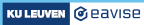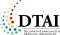3.4. FO in FO(·): Typical uses of quantifiers

### 3.4 Typical uses of quantifiers

There are a number of often-occurring patterns of knowledge, for which it is useful to know how to express them. To illustrate the first one, we return to the Wason selection task introduced above. The following structure represents a set of four cards, of which we know both sides. The theory expresses the rule of the task: "if there is a D on one side, then there is a 3 on the other side." First, try to figure out yourself whether these four cards satisfy this rule. Then, click the run button to verify.

 D
 K
 A
 D

The general pattern of the knowledge that is represented here is as follows:

• In English: For each SOMETHING which satisfies CONDITION1, it is the case that CONDITION2
• In logic: `!x in SOMETHING: CONDITION1 => CONDITIONS2`

For the above example, this is:

• For each card such that there is a D on the front of the card, it is the case that there is a 3 on the back of the card
• `!x in Card: frontSide(c) = D => backSide(c) = 3.`

Make sure that you understand why the meaning of the logic formula coincides with that of the English sentence. One way of doing this is to go over each of the four cards in the example and double-check if they satisfy the implication. This combination of a universal quantifier `!` and an implication `=>` occurs very often in practice.

### Exercise

The above structure can be turned into a model of the theory by changing a single value on one of the cards. In fact, there are two different ways of doing this. What are they? You can try out your answer above to see if you are correct.

Our theory above has as its models precisely those structures in which the Wason rule holds. We now want to write a theory with the opposite meaning, i.e., a theory that has as its models those structures in which the Wason rule does not hold. As we know from our earlier discussion of the experiment, to demonstrate that the rule does not hold, it suffices (and is also necessary) to find a card that has a D on one side and something other than a 3 on the other side. The following theory expresses that there is such a card.

```theory NonWasonT: WasonV {
?c in Card: frontSide(c) = D & backSide(c) ~= 3.
}
```

Here, the knowledge pattern that we represent is:

• In English: There is a SOMETHING which satisfies CONDITION1 such that CONDITION2.
• In logic: `?x in SOMETHING: CONDITION1 & CONDITION2.`

For the above example, this is:

• There is a card which has a D on the front such that there is something other than a 3 on the back.
• `?x in Card: frontSide(c) = D & backSide(c) ~= 3.`

This combination of an existential quantifier `?` and a conjunction `&` is a second pattern that occurs often in practice.

In the above example, we were looking for formulas whose models would be precisely the non-models of our original Wason formula. Could we not have done this by simply placing a negation `~` in front of our original formula? In other words, how about this theory?

```theory NonWasonT2: WasonV {
~(!c in Card: frontSide(c) = D => backSide(c) ~= 3).
}
```

This is indeed also a correct solution, since this theory is equivalent to `NonWasonT2`. (Here, "equivalent to" means that both theories have precisely the same models.). Indeed, we saw earlier that `~(!x: F)` is equivalent to `?x:~F`, that `F => G` is equivalent to `~F | G` and that `~(F | G)` is equivalent to `~F & ~G`. Using those three equivalences, it is easy to demonstrate the equivalence of `NonWasonT` and `NonWasonT2`:

• `~(!c in Card: frontSide(c) = D => backSide(c) = 3)`
• `~(!c in Card: frontSide(c) ~= D | backSide(c) = 3)`
• `?c in Card: ~(frontSide(c) ~= D | backSide(c) = 3)`
• `?c in Card: (frontSide(c) = D & backSide(c) ~= 3)`

### Exercise

Our earlier representation of the `LESS+FOOD=DIET` letter puzzle represented each letter by a constant of type `() -> Digit`. The vocabulary below uses a different representation, in which each letter corresponds to a value of type `Letter`. The fact that the letters are now values, rather than constants, allows us to use quantifiers to state properties of all letters. In this way, we can avoid the long-winded formula from our original representation.

Note that we enumerate the values of the type `Letter` in the vocabulary (instead of in a structure) to be able to use these symbols (L, E, S, ...) in the theory. Note also that we now write `valueOf(L)` instead of `L()` as with our previous vocabulary.

We have already prepared the formula expressing that the equation `LESS+FOOD=DIET` must hold. Previously, we mentioned that there are two additional rules present in letter puzzles.

• The first letter of each word cannot be 0.
• Each number can only be used by one letter.
Try to express these rules with what we've learned so far. Tip: use the `startsWord` relation to know which letters are at the beginning of a word. Tip2: if you quantify twice over `Letter` for the second rule, don't forget that you will also compare each letter to itself.

The expression `!x, y in Letter: F` is simply a shorthand for a double quantifier `!x in Letter: !y in Letter: F`.

### Exercise

The graph colouring problem is the problem is assigning a colour to each node in a graph, such that adjacent nodes (i.e., nodes with an edge between them) have a different colour. Add a formula that represents this constraint to following theory.

This theory can then be used to compute the different ways of colouring a given graph: if we represent the given graph by a structure that enumerates the type Node and relation edge, the models of the theory that extend this structure are precisely that valid colourings of the graph.

###### Made with love (and Hugo) by Joost Vennekens and Simon Vandevelde. :-)